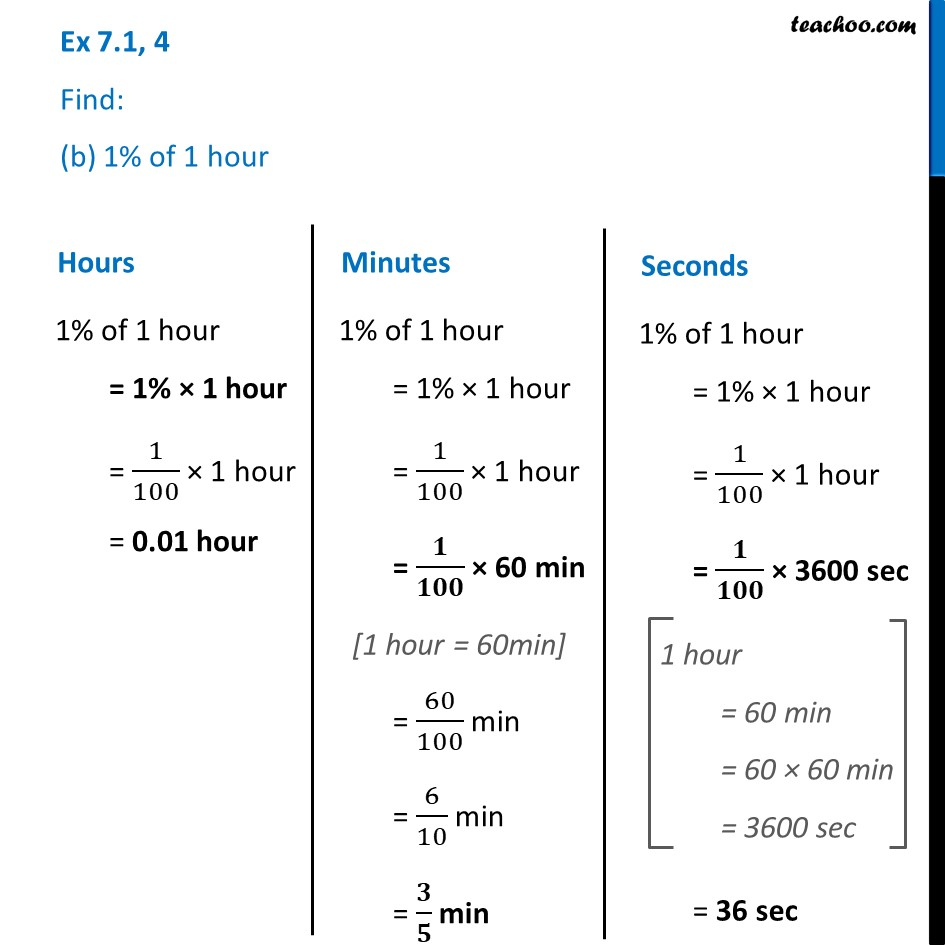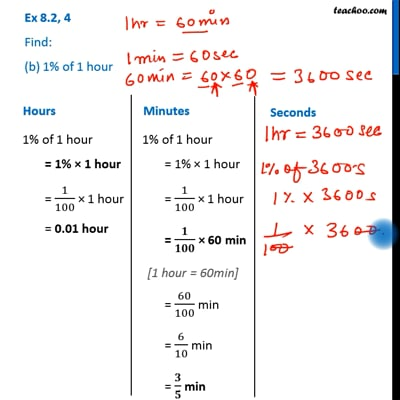Ex 7.1

Chapter 7 Class 7 Comparing Quantities
Serial order wiseThis video is only available for Teachoo black users

Learn in your speed, with individual attention - Teachoo Maths 1-on-1 Class

### Transcript

Ex 7.1, 4 Find: (b) 1% of 1 hour 1% of 1 hour = 1% × 1 hour = 1/100 × 1 hour = 0.01 hour 1% of 1 hour = 1% × 1 hour = 1/100 × 1 hour = 𝟏/𝟏𝟎𝟎 × 60 min = 60/100 min = 6/10 min = 𝟑/𝟓 min 1% of 1 hour = 1% × 1 hour = 1/100 × 1 hour = 𝟏/𝟏𝟎𝟎 × 3600 sec = 36 sec